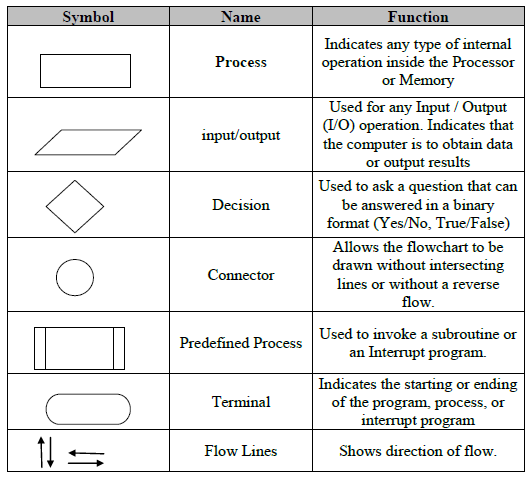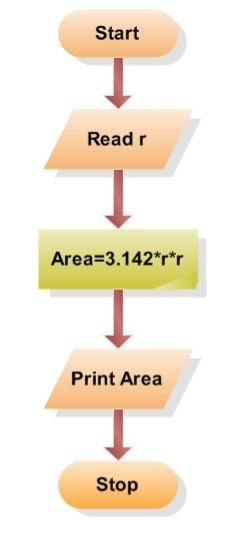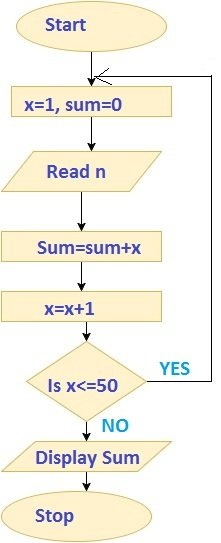What is algorithm and flowchart?

Algorithm and flowchart are programming tools. A Programmer uses various programming languages to create programs. But before actually writing a program in a programming language, a programmer first needs to find a procedure for solving the problem which is known as planning the program. The program written without proper pre-planning have higher chances of errors. The tools that are used to plan or design the problem are known as programming tools. Algorithm and flowchart are widely used programming tools.Algorithm:

The word “algorithm” relates to the name of the mathematician Al-khowarizmi, which means a procedure or a technique. Programmer commonly uses an algorithm for planning and solving the problems.

An algorithm is a specific set of meaningful instructions written in a specific order for carrying out or solving a specific problem.

Types of Algorithm:

The algorithm and flowchart are classified into three types of control structures.

1. Sequence
2. Branching(Selection)
3. Loop(Repetition)

According to the condition and requirement, these three control structures can be used.

In the sequence structure, statements are placed one after the other and the execution takes place starting from up to down.

Whereas in branch control, there is a condition and according to a condition, a decision of either TRUE or FALSE is achieved. In the case of TRUE, one of the two branches is explored; but in the case of FALSE condition, the other alternative is taken. Generally, the ‘IF-THEN’ is used to represent branch control.

Example:

Write an algorithm to find the smallest number between two numbers

Step1: Start

Step2: Input two numbers, say A and B

Step3: If A<B then small = A

Step4: If B<A then Small = B

Step5: Print Small

Step 6: End

Write an algorithm to check odd or even number

Step1:  Start

Step2: Read/Input a number and store in A

Step3: Is A<0?

If  YES then C=”ODD”

If NO then c=”even”

Step4: Display C

Step5: Stop

The Loop or Repetition allows a statements or block of statements to be executed repeatedly based on certain loop condition. ‘While’ and ‘for’ construct are used to represent the loop structure in most programming languages. Loops are of two types: Bounded and Unbounded loop. In bounded loop, the number of iterations is fixed while in unbounded loops the condition has to satisfy to end the loop.

Examples:

An algorithm to calculate even numbers between 20 and 40

Step1: Start

Step2: Store 20 in I

Step3: Display I

Step4: Add 2 to I

Step5: IF(I<=40) then go to Step 3

Step6: End

Write an algorithm to input a natural number, n, and calculate the odd numbers equal or less than n.

Step1: Start

Step2: Read a number say n

Step3: Store 1 in I

Step4: Display I

Step5: Add 2 in I

Step6: IF(I<=n) then go to line 4

Step6: End

Characteristics of a good algorithm.

1. The Finite number of steps:

After starting an algorithm for any problem, it has to terminate at some point.

1. Easy Modification.

There can be numbers of steps in an algorithm depending on the type of problem. It supports easy modification of Steps.

1. Easy and simple to understand

A Simple English language is used while writing an algorithm. It is not dependent on any particular programming language. People without the knowledge of programming can read and understand the steps in the algorithm.

1. Output

An algorithm is just a design of a program. Every program needs to display certain output after processing the input data. So one always expects the result as an output from an algorithm. It can give output at different stages. The result obtained at the end of an algorithm is known as an end result and if the result is obtained at an intermediate stage of process or operation then the result is known as an intermediate result. Also, the output has to be as expected having some relation to the inputs.

Flowchart:

The first design of flowchart goes back to 1945 which was designed by John Von Neumann. Unlike an algorithm, Flowchart uses different symbols to design a solution to a problem. It is another commonly used programming tool.

In general, a flowchart is a diagram that uses different symbols to visually present the flow of data. By looking at a flow chart one can understand the operations and sequence of operations performed in a system. This is why flowchart is often considered as a blueprint of a design used for solving a specific problem.

A flowchart is defined as a symbolic or a graphical representation of an algorithm that uses different standard symbols.

Flowchart Symbols:Guidelines for drawing a flowchart.

1. The Title for every flowchart is compulsory.
2. There must be START and END point for every flowchart.
3. The symbols used in flowchart should have only one entry point on the top. The exit point for symbols (except for decision/diamond symbol) is on the button.
4. There should be two exit points for decision symbol; exit points can be on the bottom and one side or on the sides.
5. The flow of flowchart is generally from top to bottom. But in some cases, it can also flow to upward direction
6. The direction of the flow of control should be indicated by arrowheads.
7. The operations for every step should be written inside the symbol.
8. The language used in flowchart should be simple so that it can be easily understood.
9. The flowlines that show the direction of flow of flowchart must not cross each other.
10. While connecting different pages of the same flowchart, Connectors must be used.

Some examples of algorithm and flowchart.

Example1: To calculate the area of a circle

Algorithm:

Step1: Start

Step2: Input radius of the circle say r

Step3: Use the formula πr2 and store result in a variable AREA

Step4: Print AREA

Step5: Stop

Flowchart:Example 2: Design an algorithm and flowchart to input fifty numbers and calculate their sum.

Algorithm:

Step1: Start

Step2: Initialize the count variable to zero

Step3: Initialize the sum variable to zero

Step4: Read a number say x

Step 5: Add 1 to the number in the count variable

Step6: Add the number x to the sum variable.

Step7: Is the count variable in the memory greater than 50?

If yes, display the sum: go to step 8.

If No, Repeat from step 4
Step8: Stop

Flowchart